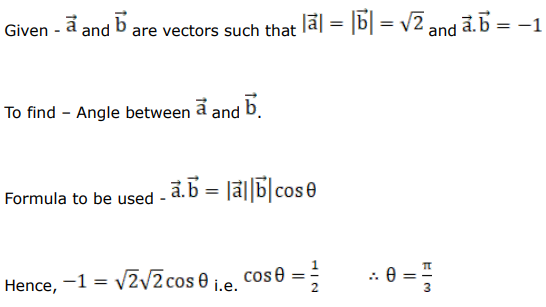# Mark against the correct answer in each of the following:

Question:

Mark $(\sqrt{)}$ against the correct answer in each of the following:

If $\vec{a}$ and $\vec{b}$ are two vectors such that $|\vec{a}|=|\vec{b}|=\sqrt{2}$ and $\vec{a} \cdot \vec{b}=-1$ then the angle between

$\vec{a}$ and $\vec{b}$ is

A. $\frac{\pi}{6}$

B. $\frac{\pi}{4}$

C. $\frac{\pi}{3}$

D. $\frac{2 \pi}{3}$

Solution: